# 1 Problem Statement

This vignette is designed to help you think about the problem of trajectory inference in the presence of two or more conditions. These conditions may be thought of as an organism-level status with potential effects on the lineage topology, ie. “case vs. control” or “mutant vs. wild-type.” While some such conditions may induce global changes in cell composition or cell state, we will assume that at least some cell types along the trajectory of interest remain comparable between conditions.

# 2 Methods

While it may seem reasonable to perform trajectory inference separately on cells from our different conditions, this is problematic for two reasons: 1) inferred trajectories will be less stable, as they would use only a subset of the available data, and 2) there would be no straightforward method for combining these results (eg. how would we map a branching trajectory onto a non-branching trajectory?).

Therefore, we propose the following workflow: first, use all available cells to construct the most stable trajectory possible. Second, use this common trajectory framework to compare distributions of cells from different conditions. We will demonstrate this process on an example dataset.

data('slingshotExample')
rd <- slingshotExample$rd cl <- slingshotExample$cl
condition <- factor(rep(c('A','B'), length.out = nrow(rd)))
condition[110:140] <- 'A'
ls()
##  "cl"               "condition"        "rd"               "slingshotExample"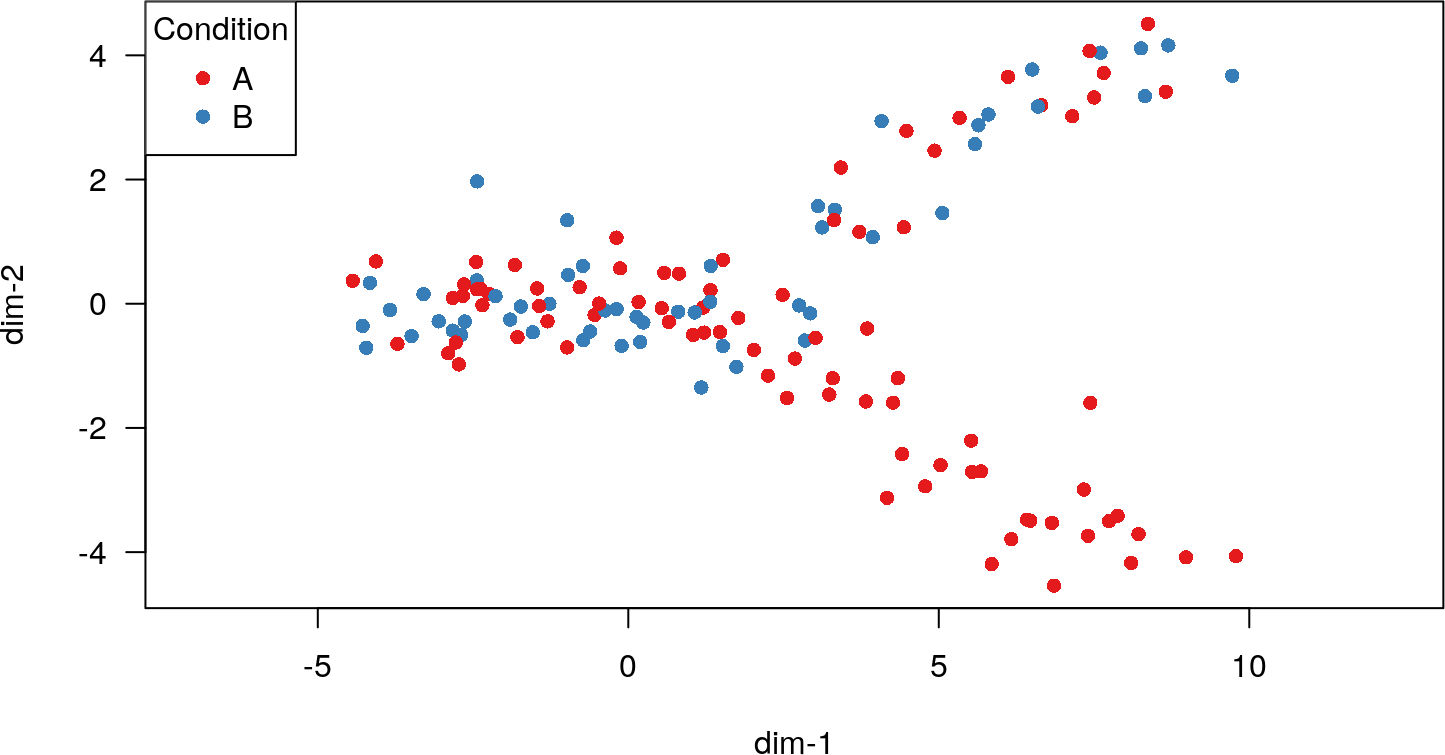This dataset consists of a branching trajectory with two conditions (A and B). Under condition A, we find cells from all possible states along the trajectory, but under condition B, one of the branches is blocked and only one terminal state can be reached.

## 2.1 Trajectory Inference

We will start by performing trajectory inference on the full dataset. In principle, any method could be used here, but we will use slingshot (cf. PopGenGoogling).

sds <- slingshot(rd, cl)
## Using full covariance matrix
## Warning in if (class(X) == "dist") X <- as.matrix(X): the condition has length >
## 1 and only the first element will be used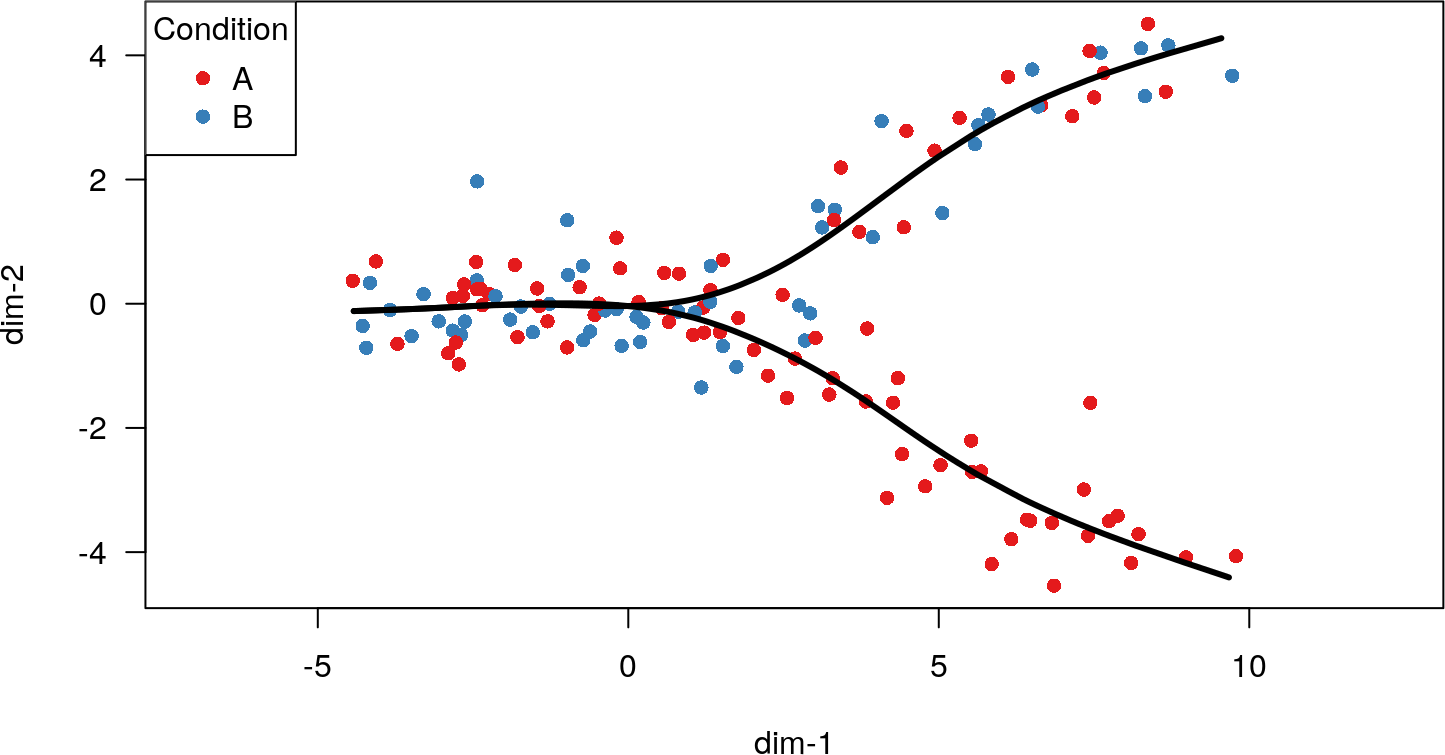Each cell has now been assigned to one or two lineages (with an associated weight indicating the certainty of each assignment) and pseudotime values have been calculated.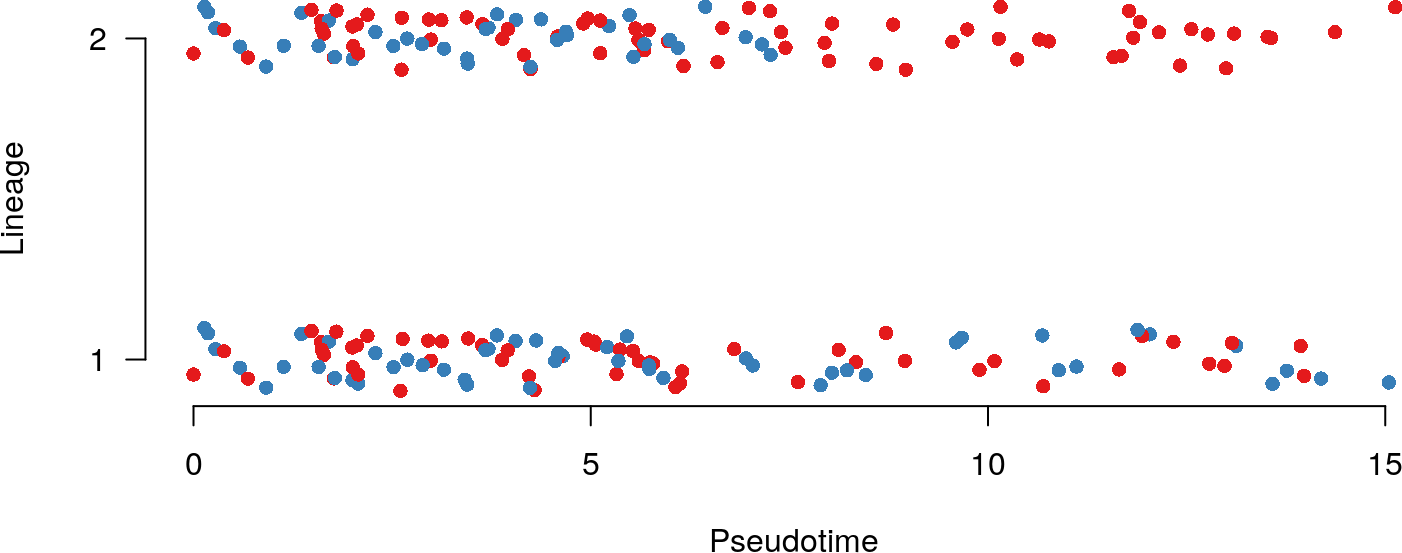## 2.2 Approach 1: Distributional Differences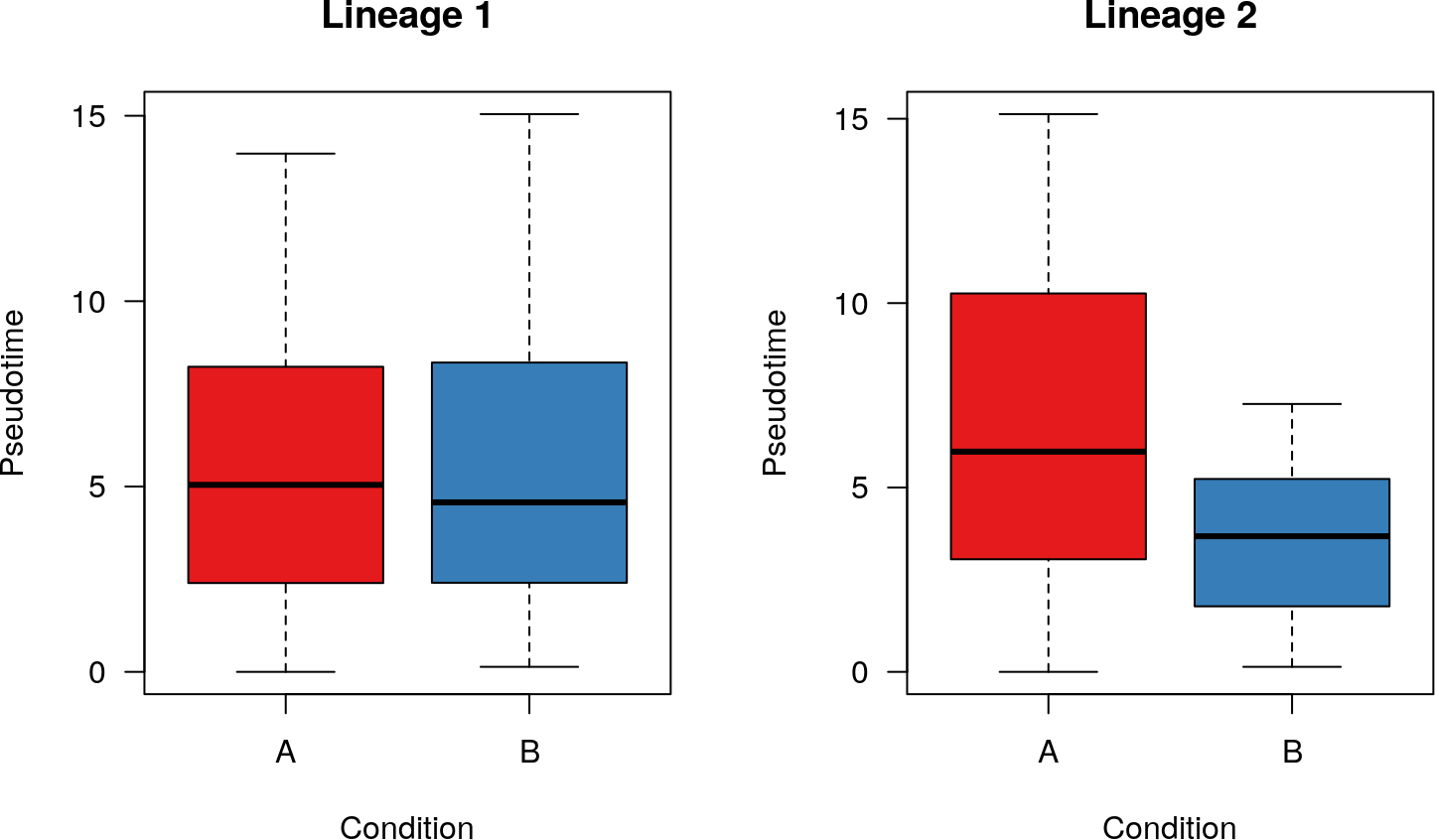Now that we have the trajectory, we are interested in testing for differences between the conditions. Any difference in the distributions of cells along a lineage may be meaningful, as it may indicate an overabundance of a particular cell type(s) in one condition. Thus, for this paradigm, we ultimately recommend a Kolmogorov-Smirnov test for detecting differences in the distributions of cells along a lineage. However, we will begin with an illustrative example of testing for a location shift.

### 2.2.1 Permutation Test

A T-test would work for this, but we’ll use a slightly more robust permutation test. Specifically, we will look for a difference in the weighted means of the pseudotime values between the two conditions.

# Permutation test
t1 <- slingPseudotime(sds, na=FALSE)[,1]
w1 <- slingCurveWeights(sds)[,1]
d1 <- weighted.mean(t1[condition=='A'], w1[condition=='A']) -
weighted.mean(t1[condition=='B'], w1[condition=='B'])
dist1 <- replicate(1e4, {
condition.i <- sample(condition)
weighted.mean(t1[condition.i=='A'], w1[condition.i=='A']) -
weighted.mean(t1[condition.i=='B'], w1[condition.i=='B'])
})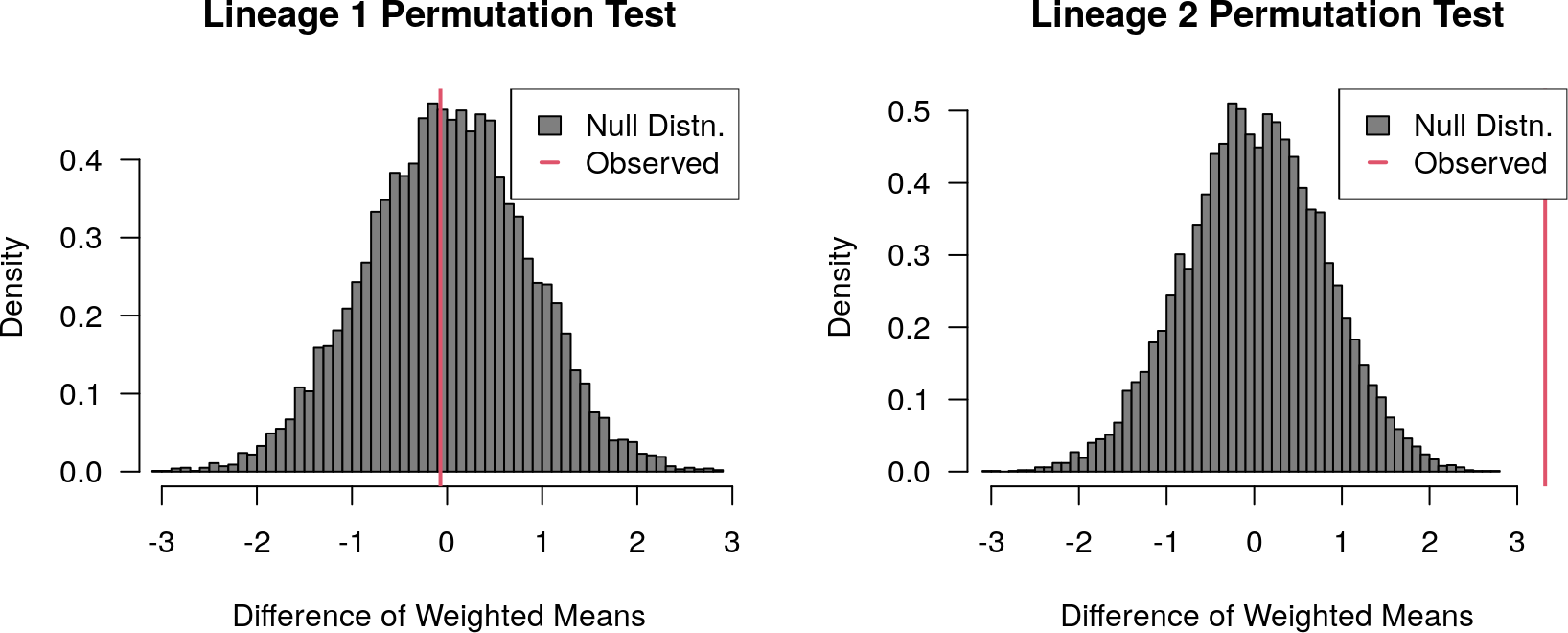paste0('Lineage 1 p-value: ', mean(abs(dist1) > abs(d1)))
##  "Lineage 1 p-value: 0.9347"
paste0('Lineage 2 p-value: ', mean(abs(dist2) > abs(d2)))
##  "Lineage 2 p-value: 0"

As we would expect, we see a significant difference in the second lineage (where condition B is impeded), but not in the first. However, because the two conditions have different distributions along the second lineage, the exchangeability assumption is violated and the resulting p-value is not valid.

### 2.2.2 Kolmogorov-Smirnov Test

Another, more general approach we could take to test for a difference in distributions would be the Kolmogorov-Smirnov test (or a similar permutation test using that test’s statistic). This test would, in theory, allow us to pick up subtler differences between the conditions, such as an overabundance of a cell type in one condition.

# Kolmogorov-Smirnov test
ks.test(slingPseudotime(sds)[condition=='A',1], slingPseudotime(sds)[condition=='B',1])
##
##  Two-sample Kolmogorov-Smirnov test
##
## data:  slingPseudotime(sds)[condition == "A", 1] and slingPseudotime(sds)[condition == "B", 1]
## D = 0.081119, p-value = 0.9852
## alternative hypothesis: two-sided
ks.test(slingPseudotime(sds)[condition=='A',2], slingPseudotime(sds)[condition=='B',2])
##
##  Two-sample Kolmogorov-Smirnov test
##
## data:  slingPseudotime(sds)[condition == "A", 2] and slingPseudotime(sds)[condition == "B", 2]
## D = 0.42254, p-value = 0.0001851
## alternative hypothesis: two-sided

Again, we see a significant difference in the second lineage, but not in the first. Note that unlike the difference of weighted means we used above, this test largely ignores the weights which assign cells to lineages, using any cell with a lineage weight greater than 0 (those with weights of zero are assigned pseudotime values of NA). Theoretically, one could use weights with the Kolmogorov-Smirnov test, as the test statistic is based on the maximum difference between cumulative distribution functions, but we were unable to find an implementation of this in base R or the stats package. For more stringent cutoffs, the user could specify a minimum lineage weight, say of 0.5. Due to this test’s ability to detect a wide variety of differences, we would recommend it over the previous procedure for general purpose use.

## 2.3 Approach 2: Condition Imbalance

Below, the problem is somewhat rephrased as compared to the approaches used above. Whereas the permutation and KS tests were testing within-lineage differences in pseudotime values between conditions, the approaches suggested below rather test for within-lineage condition imbalance along the progression of pseudotime.

The advantages of this approach would be:

• more straightforward comparison between lineages.
• identification of pseudotime regions where the distribution of conditions is skewed.
• automatic adjustment for baseline condition probabilities as determined by the inception of the trajectory (for example, if one condition naturally has 80% of cells and the other has 20%, which could then evolve to a different proportion as pseudotime progresses). Note that the approaches above could also handle this, but they do not directly estimate a change in contribution for the conditions (e.g. you won’t be able to say that you went from 75% condition A to 50% condition A, only that something’s happened).

Its disadvantages, however, are:

• requiring explicit distributional assumptions.
• possibly more difficult to extend to assessing shifts in other moments than the mean (e.g. variance).

### 2.3.1 Logistic Regression

As a first approach, one might check whether the probability of having a cell from a particular condition changes across pseudotime in any lineage. For two conditions, this may proceed using binomial logistic regression, which might be extended to multinomial logistic regression for multiple conditions. To loosen the restrictive binomial assumption, we allow for a more flexible variance structure by using a quasibinomial model.

pt <- slingPseudotime(sds, na=FALSE)
cw <- slingCurveWeights(sds)
assignment <- apply(cw, 1, which.max)
ptAs <- c() #assigned pseudotime
for(ii in 1:nrow(pt)) ptAs[ii] <- pt[ii,assignment[ii]]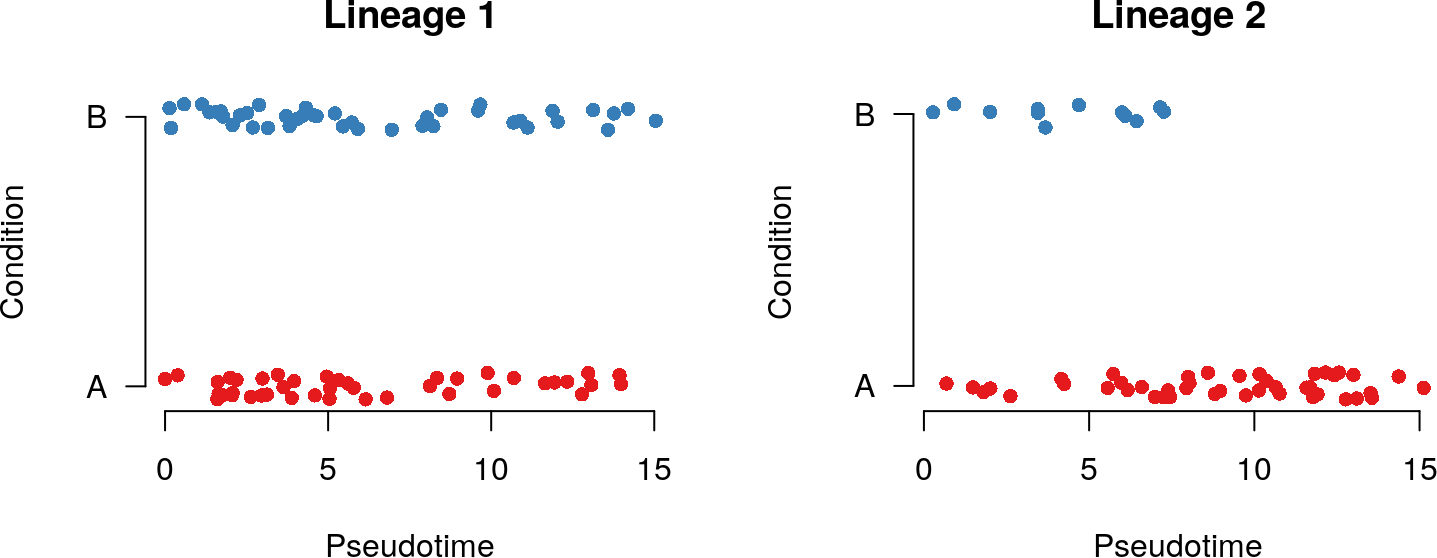# model for lineage 1: not significant
cond1 <- factor(condition[assignment == 1])
t1 <- ptAs[assignment == 1]
m1 <- glm(cond1 ~ t1, family = quasibinomial(link = "logit"))
summary(m1)
##
## Call:
## glm(formula = cond1 ~ t1, family = quasibinomial(link = "logit"))
##
## Deviance Residuals:
##      Min        1Q    Median        3Q       Max
## -1.18654  -1.17608  -0.00156   1.17872   1.18450
##
## Coefficients:
##              Estimate Std. Error t value Pr(>|t|)
## (Intercept) -0.017048   0.389350  -0.044    0.965
## t1           0.002756   0.051836   0.053    0.958
##
## (Dispersion parameter for quasibinomial family taken to be 1.02439)
##
##     Null deviance: 116.45  on 83  degrees of freedom
## Residual deviance: 116.45  on 82  degrees of freedom
## AIC: NA
##
## Number of Fisher Scoring iterations: 3
# model for lineage 2: significant
cond2 <- factor(condition[assignment == 2])
t2 <- ptAs[assignment == 2]
m2 <- glm(cond2 ~ t2, family = quasibinomial(link = "logit"))
summary(m2)
##
## Call:
## glm(formula = cond2 ~ t2, family = quasibinomial(link = "logit"))
##
## Deviance Residuals:
##     Min       1Q   Median       3Q      Max
## -1.5393  -0.5988  -0.3347  -0.1932   1.8858
##
## Coefficients:
##             Estimate Std. Error t value Pr(>|t|)
## (Intercept)   1.0700     0.6403   1.671 0.100475
## t2           -0.3666     0.1021  -3.591 0.000712 ***
## ---
## Signif. codes:  0 '***' 0.001 '**' 0.01 '*' 0.05 '.' 0.1 ' ' 1
##
## (Dispersion parameter for quasibinomial family taken to be 0.744841)
##
##     Null deviance: 58.193  on 55  degrees of freedom
## Residual deviance: 44.309  on 54  degrees of freedom
## AIC: NA
##
## Number of Fisher Scoring iterations: 5

### 2.3.2 Additive Logistic Regression

Advantages:

• By extending the linearity assumption, this approach can detect more subtle patterns, e.g. intermediate regions of a lineage that lack cells from a particular condition. This would be reflected by a bimodal distribution of pseudotimes for that condition.
• Also easily extends to multiple conditions using multinomial additive models.
### note that logistic regression is monotone hence only allows for increasing or decreasing proportion of cells for a particular condition.
### hence, for complex trajectories, you could consider smoothing the pseudotime, i.e.
m1s <- mgcv::gam(cond1 ~ s(t1), family="quasibinomial")
summary(m1s)
##
## Family: quasibinomial
## Link function: logit
##
## Formula:
## cond1 ~ s(t1)
##
## Parametric coefficients:
##              Estimate Std. Error t value Pr(>|t|)
## (Intercept) 6.526e-08  2.209e-01       0        1
##
## Approximate significance of smooth terms:
##       edf Ref.df     F p-value
## s(t1)   1      1 0.003   0.958
##
## R-sq.(adj) =  -0.0122   Deviance explained = 0.00249%
## GCV = 1.4547  Scale est. = 1.0244    n = 84
m2s <- mgcv::gam(cond2 ~ s(t2), family="quasibinomial")
summary(m2s)
##
## Family: quasibinomial
## Link function: logit
##
## Formula:
## cond2 ~ s(t2)
##
## Parametric coefficients:
##             Estimate Std. Error t value Pr(>|t|)
## (Intercept)   -3.349      1.898  -1.765   0.0834 .
## ---
## Signif. codes:  0 '***' 0.001 '**' 0.01 '*' 0.05 '.' 0.1 ' ' 1
##
## Approximate significance of smooth terms:
##         edf Ref.df     F p-value
## s(t2) 2.751  3.456 1.337   0.325
##
## R-sq.(adj) =  0.244   Deviance explained = 33.2%
## GCV = 0.79718  Scale est. = 1.0718    n = 56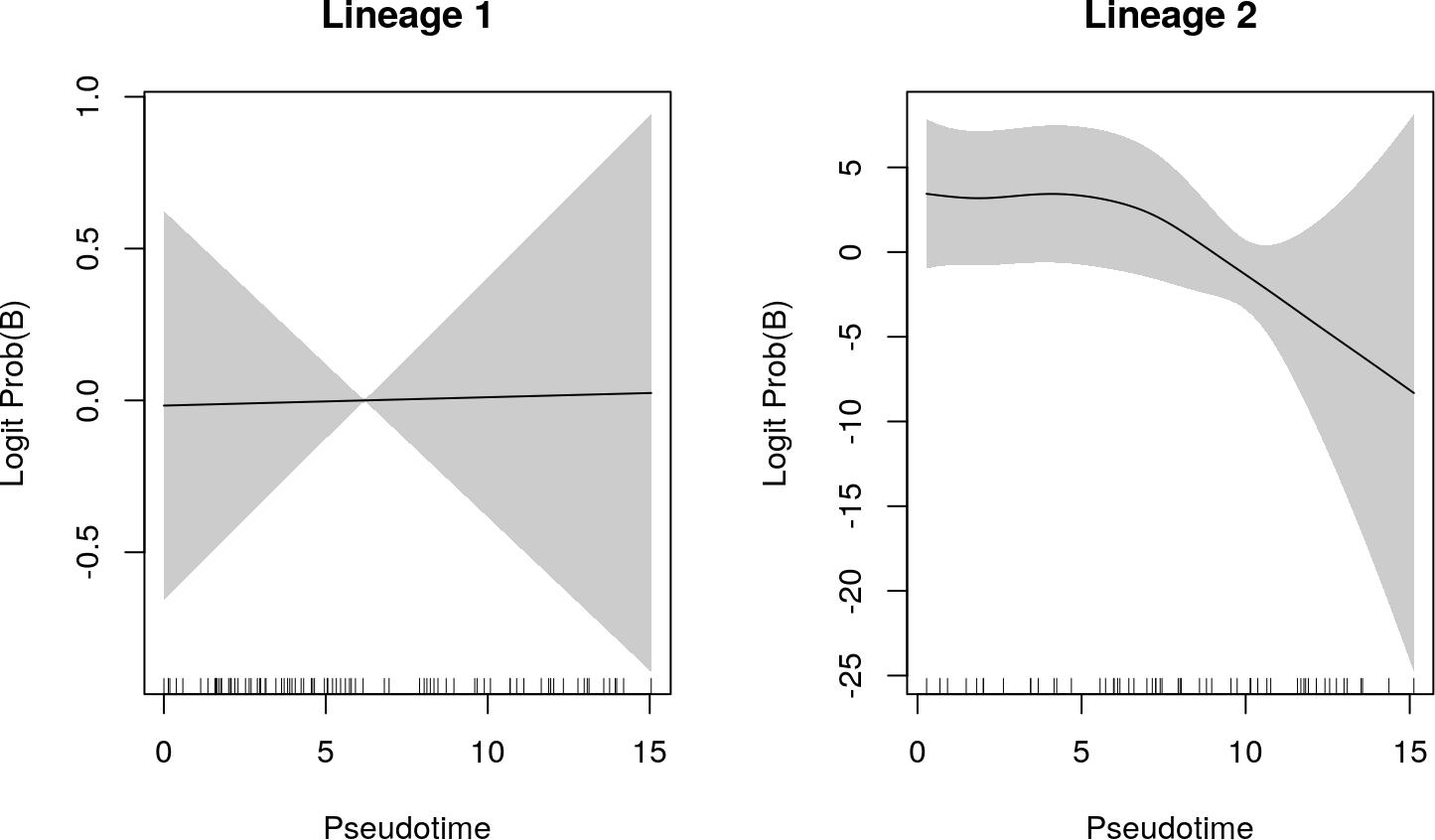### 2.3.3tradeSeq-like

With apologies, suppose that the probability of a particular condition decreases in both lineages, but moreso in one than the other. This can be obscured with the above approaches, especially if the lineages have different lengths. In that case, it would be informative to compare the lineages directly. We can accomplish this goal with an additive model.

The code below fits an additive model with smoothing terms for the pseudotimes along each lineage. The smoothers may be directly compared since we require the same penalization and basis functions (by setting id = 1). Based on the fitted model, inference would be exactly like we do in tradeSeq.

require(mgcv, quietly = TRUE)
## This is mgcv 1.8-31. For overview type 'help("mgcv-package")'.
t1 <- pt[,1]
t2 <- pt[,2]
l1 <- as.numeric(assignment == 1)
l2 <- as.numeric(assignment == 2)
m <- gam(condition ~ s(t1, by=l1, id=1) + s(t2, by=l2, id=1),
family = quasibinomial(link = "logit"))
summary(m)
##
## Family: quasibinomial
## Link function: logit
##
## Formula:
## condition ~ s(t1, by = l1, id = 1) + s(t2, by = l2, id = 1)
##
## Parametric coefficients:
##             Estimate Std. Error t value Pr(>|t|)
## (Intercept)  -1.1951     0.3935  -3.037  0.00286 **
## ---
## Signif. codes:  0 '***' 0.001 '**' 0.01 '*' 0.05 '.' 0.1 ' ' 1
##
## Approximate significance of smooth terms:
##          edf Ref.df     F p-value
## s(t1):l1   2      2 3.504  0.0327 *
## s(t2):l2   1      1 9.327  0.0027 **
## ---
## Signif. codes:  0 '***' 0.001 '**' 0.01 '*' 0.05 '.' 0.1 ' ' 1
##
## Rank: 20/21
## R-sq.(adj) =   0.12   Deviance explained = 13.9%
## GCV = 1.2168  Scale est. = 1.0294    n = 140
### and then we're back to tradeSeq-like inference ...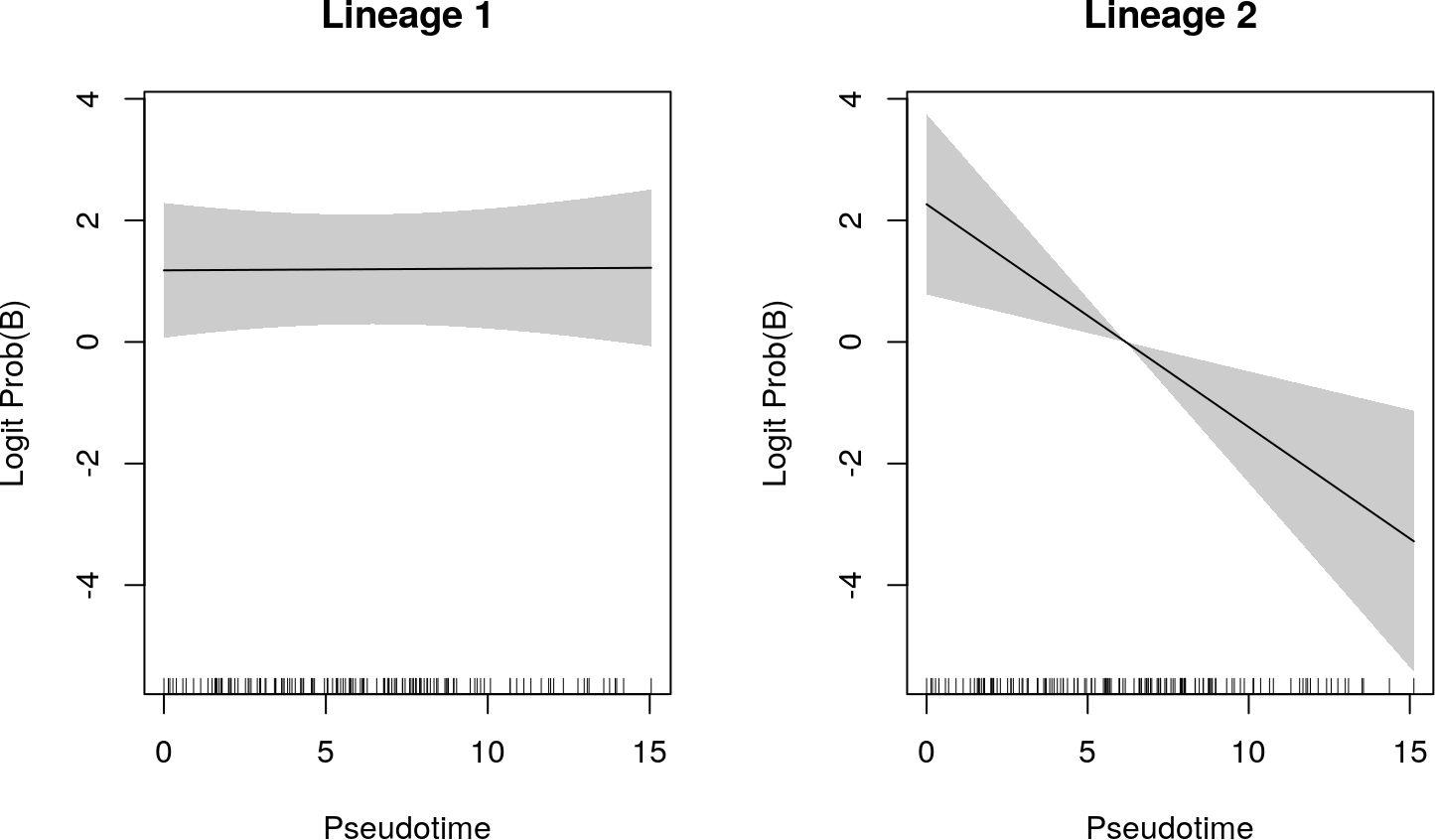## 2.4 Other Approaches

The frameworks suggested here are only a few of the many possible approaches to a highly complex problem.

We can envision another potential approach, similar to the Condition Imbalance framework, but requiring fewer parametric assumptions, inspired by the batch effect metric of Büttner, et al. (2019) (see Figure 1). Essentially, we may be able to march along the trajectory and check for condition imbalance in a series of local neighborhoods, defined by the k nearest neighbors of particular cells.

# 3 Parting Words

For both of the above procedures, it is important to note that we are making multiple comparisons (in this case, 2). The p-values we obtain from these tests should be corrected for multiple testing, especially for trajectories with a large number of lineages.

That said, trajectory inference is often one of the last computational methods in a very long analysis pipeline (generally including gene-level quantification, gene filtering / feature selection, and dimensionality reduction). Hence, we strongly discourage the reader from putting too much faith in any p-value that comes out of this analysis. Such values may be useful suggestions, indicating particular features or cells for follow-up study, but should not be treated as meaningful statistical quantities.

Finally, we would like to emphasize that these procedures are merely suggestions which have not (yet) been subjected to extensive testing and revision. If you have any ideas, questions, or results that you are willing to share, we would love to hear them! Feel free to email Kelly Street or open an issue on either the slingshot repo or tradeSeq repo on GitHub.

# 4 Session Info

sessionInfo()
## R version 4.0.0 (2020-04-24)
## Platform: x86_64-pc-linux-gnu (64-bit)
## Running under: Ubuntu 18.04.4 LTS
##
## Matrix products: default
## BLAS:   /home/biocbuild/bbs-3.11-bioc/R/lib/libRblas.so
## LAPACK: /home/biocbuild/bbs-3.11-bioc/R/lib/libRlapack.so
##
## locale:
##   LC_CTYPE=en_US.UTF-8       LC_NUMERIC=C
##   LC_TIME=en_US.UTF-8        LC_COLLATE=C
##   LC_MONETARY=en_US.UTF-8    LC_MESSAGES=en_US.UTF-8
##   LC_PAPER=en_US.UTF-8       LC_NAME=C
##   LC_ADDRESS=C               LC_TELEPHONE=C
##  LC_MEASUREMENT=en_US.UTF-8 LC_IDENTIFICATION=C
##
## attached base packages:
##  stats     graphics  grDevices utils     datasets  methods   base
##
## other attached packages:
##  mgcv_1.8-31        nlme_3.1-148       RColorBrewer_1.1-2 slingshot_1.6.1
##  princurve_2.1.4    BiocStyle_2.16.0
##
## loaded via a namespace (and not attached):
##   Rcpp_1.0.4.6                compiler_4.0.0
##   BiocManager_1.30.10         GenomeInfoDb_1.24.2
##   XVector_0.28.0              bitops_1.0-6
##   tools_4.0.0                 zlibbioc_1.34.0
##   SingleCellExperiment_1.10.1 digest_0.6.25
##  evaluate_0.14               lattice_0.20-41
##  pkgconfig_2.0.3             rlang_0.4.6
##  Matrix_1.2-18               igraph_1.2.5
##  DelayedArray_0.14.0         magick_2.4.0
##  yaml_2.2.1                  parallel_4.0.0
##  xfun_0.15                   GenomeInfoDbData_1.2.3
##  stringr_1.4.0               knitr_1.29
##  S4Vectors_0.26.1            IRanges_2.22.2
##  stats4_4.0.0                grid_4.0.0
##  Biobase_2.48.0              rmarkdown_2.3
##  bookdown_0.20               magrittr_1.5
##  splines_4.0.0               matrixStats_0.56.0
##  htmltools_0.5.0             BiocGenerics_0.34.0
##  GenomicRanges_1.40.0        SummarizedExperiment_1.18.1
##  ape_5.4                     stringi_1.4.6
##  RCurl_1.98-1.2